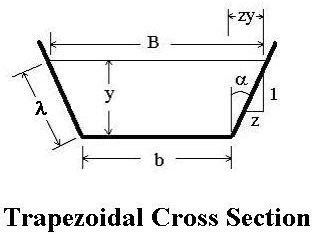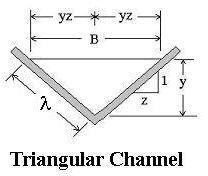# Calculation of Open Channel Flow Hydraulic Radius: Calculate using Trapezoid Area

Page content

## Introduction

One of the parameters needed in order to make use of the Manning equation for open channel flow calculations is the hydraulic radius of the channel cross section. Common shapes for open channel cross section include rectangle, trapezoid, triangle, and circle.

The use of hydraulic radius in Manning equation calculations is covered in the first article of this series, ‘Introduction to the Manning Equation for Open Channel Flow Calculations’ and the hydraulic radius and use of the Manning equation for a circular pipe are covered in ‘How to Use the Manning Equation for Storm Sewer Calculations.’

This article will cover rectangle, trapezoid, triangle, and circular shapes for an open channel cross section. Hydraulic radius is defined as the cross sectional area of flow divided by the wetted perimeter, so the calculation of rectangle and trapezoid area and triangle area will be included along with the perimeter for each.

## Rectangular Cross Section

The simplest open channel flow cross section for calculation of hydraulic radius is a rectangle. The depth of flow is often represented by the symbol, y, and b is often used for the channel bottom width, as shown in the diagram at the left. From the hydraulic radius definition: RH = A/P, where A is the cross sectional area of flow and P is its wetted perimeter. From the diagram it is clear that A = by and P = 2y + b, so the hydraulic radius is: RH = by/(2y + b) for an open channel flow through a rectangular cross section.

## Trapezoidal Cross Section

A trapezoid shape is sometimes used for manmade channels and the cross section of natural stream channels are often approximated by a trapezoid area. The diagram at the right shows a trapezoid and the parameters typically used for its shape and size in open channel flow calculations. Those parameters, which are used to calculate the trapezoid area and wetted perimeter, are y, the liquid depth; b, the bottom width; B the width of the liquid surface; λ, the wetted length measured along the sloped side; and α, the angle of the sloped side from vertical. The side slope is usually specified as horiz:vert = z:1.

The cross sectional area of flow is the trapezoid area: A = y(b + B)/2, orA = (y/2)(b + b + 2zy), because B = b + 2zy, as can be seen from the diagram.

Simplifying, the trapezoid area is: A = by + zy2.

The wetted perimeter is: P = b + 2λ, but by Pythagoras Theorem:

λ2 = y2 + (yz)2, or λ = [y2 + (yz)2]1/2, so the wetted perimeter is:

P = b + 2y(1 + z2)1/2, and the hydraulic radius for a trapezoid is:

RH = (by + zy2)/[b + 2y(1 + z2)1/2]

## Triangular Cross SectionA triangular open channel cross section is shown in the diagram at the left. The diagram shows the typical case, where the two sides are sloped at the same angle. Fewer parameters are needed for the triangular area than for the trapezoid area. The parameters, as shown in the diagram are: B, the surface width of the liquid; λ, the sloped length of the triangle side; y, the liquid depth measured from the vertex of the triangle; and the side slope specification, horiz:vert = z:1.

The triangle area is: A = By/2, but the figure shows that B = 2yz, so the triangle area becomes simply: A = y2z.

The wetted perimeter is: P = 2λ with λ2 = y2 + (yz)2. This simplifies to: P = 2[y2(1 + z2)]1/2

The hydraulic radius is thus: RH = A/P = y2z/{2[y2(1 + z2)]1/2}

## Circular Cross Section

The hydraulic radius for a circular pipe flowing full is easy to calculate. The cross-sectional area is A = πD2/4 and the wetted perimeter is P = πD. Substituting into the equation RH = A/P and simplifying the expression gives: RH = D/4. For calculation of the hydraulic radius for partially full pipe flow, see the article, “Use of Excel Spreadsheet Templates for Partially Full Pipe Flow.” Spreadsheet templates can be downloaded from this article to calculate hydraulic radius (and other parameters) for partially full pipe flow.

## References and Image Credits

References for further information:

1. Bengtson, Harlan H., Open Channel Flow I - The Manning Equation and Uniform Flow, an online, continuing education course for PDH credit.

2. Chow, V. T., Open Channel Hydraulics, New York: McGraw-Hill, 1959.

3. Munson, B. R., Young, D. F., & Okiishi, T. H., Fundamentals of Fluid Mechanics, 4th Ed., New York: John Wiley and Sons, Inc, 2002.

Images are all from reference #1

## This post is part of the series: Uniform Open Channel Flow and the Manning Equation

The Manning equation is widely used for uniform open channel flow calculations with natural or man made channels. The Manning equation is used to relate parameters like river discharge and water flow velocity to hydraulic radius, and open channel slope, size, shape, and Manning roughness.